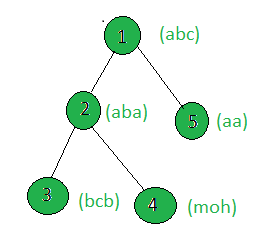# Count the nodes of the given tree whose weighted string is a palindrome

Given a tree, and the weights (in the form of strings) of all the nodes, the task is to count the nodes whose weights are palindrome.

Examples:

Input:Output: 3
Only the weights of the nodes 2, 3 and 5 are palindromes.

## Recommended: Please try your approach on {IDE} first, before moving on to the solution.

Approach: Perform dfs on the tree and for every node, check if it’s string is palindrome or not. If yes then increment the count.

Below is the implementation of the above approach:

## C++

 `// C++ implementation of the approach ` `#include ` `using` `namespace` `std; ` ` `  `int` `cnt = 0; ` ` `  `vector<``int``> graph; ` `vector weight(100); ` ` `  `// Function that returns true ` `// if x is a palindrome ` `bool` `isPalindrome(string x) ` `{ ` `    ``int` `n = x.size(); ` `    ``for` `(``int` `i = 0; i < n / 2; i++) { ` `        ``if` `(x[i] != x[n - 1 - i]) ` `            ``return` `false``; ` `    ``} ` `    ``return` `true``; ` `} ` ` `  `// Function to perform dfs ` `void` `dfs(``int` `node, ``int` `parent) ` `{ ` ` `  `    ``// Weight of the current node ` `    ``string x = weight[node]; ` ` `  `    ``// If the weight is a palindrome ` `    ``if` `(isPalindrome(x)) ` `        ``cnt += 1; ` ` `  `    ``for` `(``int` `to : graph[node]) { ` `        ``if` `(to == parent) ` `            ``continue``; ` `        ``dfs(to, node); ` `    ``} ` `} ` ` `  `// Driver code ` `int` `main() ` `{ ` ` `  `    ``// Weights of the node ` `    ``weight = ``"abc"``; ` `    ``weight = ``"aba"``; ` `    ``weight = ``"bcb"``; ` `    ``weight = ``"moh"``; ` `    ``weight = ``"aa"``; ` ` `  `    ``// Edges of the tree ` `    ``graph.push_back(2); ` `    ``graph.push_back(3); ` `    ``graph.push_back(4); ` `    ``graph.push_back(5); ` ` `  `    ``dfs(1, 1); ` ` `  `    ``cout << cnt; ` ` `  `    ``return` `0; ` `} `

## Java

 `// Java implementation of the approach  ` `import` `java.util.*; ` ` `  `class` `GFG ` `{ ` ` `  `static` `int` `cnt = ``0``;  ` ` `  `static` `Vector> graph = ``new` `Vector>();  ` `static` `Vector weight = ``new` `Vector();  ` ` `  `// Function that returns true  ` `// if x is a palindrome  ` `static` `boolean` `isPalindrome(String x)  ` `{  ` `    ``int` `n = x.length();  ` `    ``for` `(``int` `i = ``0``; i < n / ``2``; i++) ` `    ``{  ` `        ``if` `(x.charAt(i) != x.charAt(n - ``1` `- i))  ` `            ``return` `false``;  ` `    ``}  ` `    ``return` `true``;  ` `}  ` ` `  `// Function to perform dfs  ` `static` `void` `dfs(``int` `node, ``int` `parent)  ` `{  ` ` `  `    ``// Weight of the current node  ` `    ``String x = weight.get(node);  ` `     `  ` `  `    ``// If the weight is a palindrome  ` `    ``if` `(isPalindrome(x))  ` `        ``cnt += ``1``;  ` ` `  `    ``for` `(``int` `i=``0``;i()); ` `     `  `    ``// Edges of the tree  ` `    ``graph.get(``1``).add(``2``);  ` `    ``graph.get(``2``).add(``3``);  ` `    ``graph.get(``2``).add(``4``);  ` `    ``graph.get(``1``).add(``5``);  ` `    ``dfs(``1``, ``1``);  ` ` `  `    ``System.out.println( cnt);  ` `} ` `} ` ` `  `// This code is contributed by Arnab Kundu `

## Python3

 `# Python3 implementation of the approach ` `cnt ``=` `0` ` `  `graph ``=` `[``0``] ``*` `100` `for` `i ``in` `range``(``100``): ` `    ``graph[i] ``=` `[] ` ` `  `weight ``=` `[``"0"``] ``*` `100` ` `  `# Function that returns true ` `# if x is a palindrome ` `def` `isPalindrome(x): ` `    ``n ``=` `len``(x) ` ` `  `    ``for` `i ``in` `range``(``0``, n ``/``/` `2``): ` `        ``if` `x[i] !``=` `x[n ``-` `1` `-` `i]: ` `            ``return` `False` ` `  `    ``return` `True` ` `  `# Function to perform dfs ` `def` `dfs(node, parent): ` `    ``global` `cnt ` ` `  `    ``# Weight of the current node ` `    ``x ``=` `weight[node] ` ` `  `    ``# If the weight is a palindrome ` `    ``if` `(isPalindrome(x)): ` `        ``cnt ``+``=` `1` ` `  `    ``for` `to ``in` `graph[node]: ` `        ``if` `to ``=``=` `parent: ` `            ``continue` `        ``dfs(to, node) ` ` `  `# Driver Code ` `if` `__name__ ``=``=` `"__main__"``: ` ` `  `    ``# Weights of the node ` `    ``weight[``0``] ``=` `"" ` `    ``weight[``1``] ``=` `"abc"` `    ``weight[``2``] ``=` `"aba"` `    ``weight[``3``] ``=` `"bcb"` `    ``weight[``4``] ``=` `"moh"` `    ``weight[``5``] ``=` `"aa"` ` `  `    ``# Edges of the tree ` `    ``graph[``1``].append(``2``) ` `    ``graph[``2``].append(``3``) ` `    ``graph[``2``].append(``4``) ` `    ``graph[``1``].append(``5``) ` ` `  `    ``dfs(``1``, ``1``) ` ` `  `    ``print``(cnt) ` ` `  `# This code is contributed by ` `# sanjeev2552 `

## C#

 `// C# implementation of the approach  ` `using` `System; ` `using` `System.Collections.Generic;  ` ` `  `class` `GFG ` `{ ` ` `  `    ``static` `int` `cnt = 0;  ` `     `  `    ``static` `List> graph = ``new` `List>();  ` `    ``static` `List weight = ``new` `List();  ` `     `  `    ``// Function that returns true ` `    ``// if x is a palindrome ` `    ``static` `bool` `isPalindrome(``string` `x) ` `    ``{ ` `        ``int` `n = x.Length; ` `        ``for` `(``int` `i = 0; i < n / 2; i++) ` `        ``{ ` `            ``if` `(x[i] != x[n - 1 - i]) ` `                ``return` `false``; ` `        ``} ` `        ``return` `true``; ` `    ``} ` `     `  `    ``// Function to perform dfs  ` `    ``static` `void` `dfs(``int` `node, ``int` `parent)  ` `    ``{  ` `     `  `        ``// Weight of the current node  ` `        ``String x = weight[node];  ` `     `  `        ``// If the weight is a palindrome  ` `        ``if` `(isPalindrome(x))  ` `            ``cnt += 1;  ` `     `  `        ``for` `(``int` `i = 0; i < graph[node].Count; i++)  ` `        ``{  ` `            ``if` `(graph[node][i] == parent)  ` `                ``continue``;  ` `            ``dfs(graph[node][i], node);  ` `        ``}  ` `    ``}  ` `     `  `    ``// Driver code  ` `    ``public` `static` `void` `Main(String []args) ` `    ``{  ` `     `  `        ``// Weights of the node  ` `        ``weight.Add( ``""``);  ` `        ``weight.Add( ``"abc"``);  ` `        ``weight.Add( ``"aba"``);  ` `        ``weight.Add( ``"bcb"``);  ` `        ``weight.Add( ``"moh"``);  ` `        ``weight.Add( ``"aa"``);  ` `         `  `        ``for``(``int` `i = 0; i < 100; i++) ` `        ``graph.Add(``new` `List<``int``>()); ` `     `  `        ``// Edges of the tree  ` `        ``graph.Add(2);  ` `        ``graph.Add(3);  ` `        ``graph.Add(4);  ` `        ``graph.Add(5);  ` `     `  `        ``dfs(1, 1);  ` `     `  `        ``Console.WriteLine( cnt);  ` `     `  `    ``}  ` `} ` ` `  `// This code has been contributed by 29AjayKumar `

Output:

```3
```

My Personal Notes arrow_drop_up